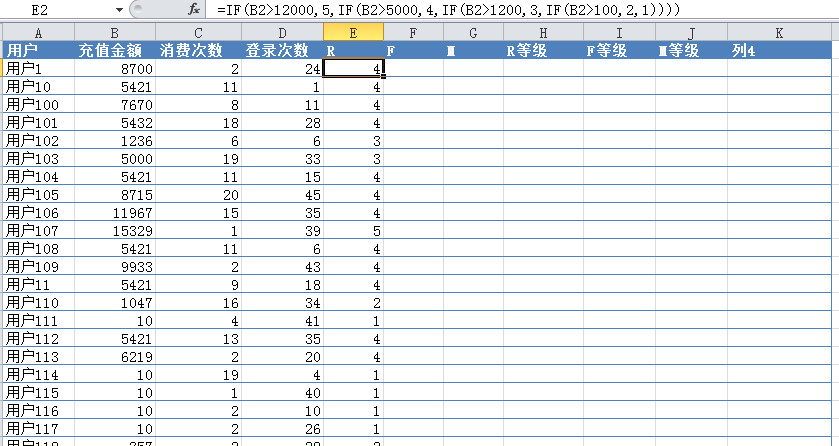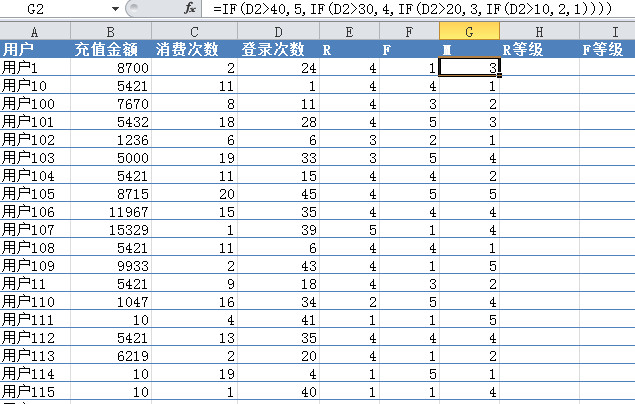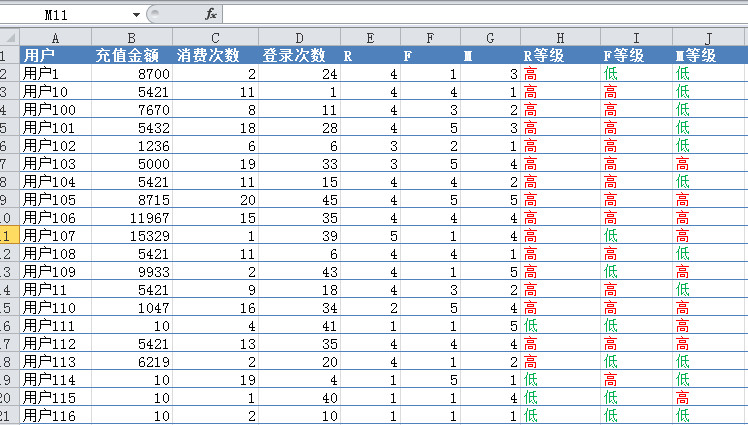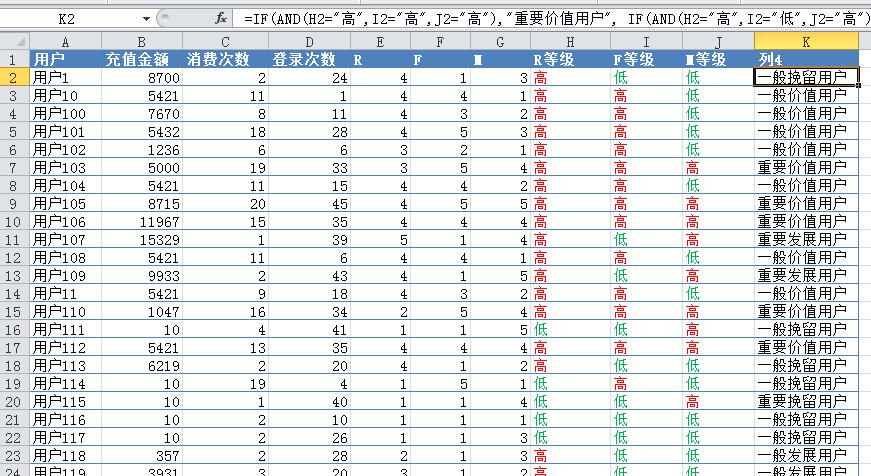# 全流程分享：利用RFM模型开展金融产品精细化运## 1. 首先根据产品确定三个重要指标

（具体的指标根据自己产品决定，如社交类产品可以是：登录次数，关注人数、使用时长）## 2. 下面开始计算R、F、M值• 1=10~100
• 2=101~1200
• 3=1201~5000
• 4=50001~12000
• 5=12001~20000

`=IF（B2>12000,5,IF（B2>5000,4,IF（B2>1200,3,IF（B2>100,2,1））））`• 1=1~5
• 2=6~7
• 3=8~10
• 4=10~15
• 5=15~20

`=IF（C2>15,5,IF（C2>10,4,IF（C2>7,3,IF（C2>5,2,1））））`

• 1=1~10
• 2=11~20
• 3=21~30
• 4=31~40
• 5=41~50

`=IF（D2>40,5,IF（D2>30,4,IF（D2>20,3,IF（D2>10,2,1））））`## 3. 求出RFM的平均值

• R的平均值=1.19
• F的平均值=1.28
• M的平均值=3.33

（有小伙伴问我，为什么要计算出数值后再求平均值，而不是在原消费金额的基础上求平均？你想下比赛时评委打分，会去掉一个最高分和最低分，这两个的道理其实有点类似，这样会使数组更加整体）

## 4. 判断RFM等级

• R=IF（E2>1.19,”高”,”低”）
• F=IF（F2>1.28,”高”,”低”）
• M=IF（G>3.33,”高”,”低”）## 5. 得出用户价值```=IF（AND（H2="高",I2="高",J2="高"）,"重要价值用户",
IF（AND（H2="高",I2="低",J2="高"）,"重要发展用户",
IF（AND（H2="低",I2="高",J2="高"）,"重要保持用户",
IF（AND（H1="低",I2="低",J2="高"）,"重要挽留用户",
IF（AND（H2="高",I2="高",J2="低"）,"一般价值用户",
IF（AND（H2="高",I2="低",J1="低"）,"一般发展用户",
IF（AND（H1="低",I2="高",J2="低"）,"一般保持用户","一般挽留用户"
）））））））```

……